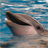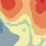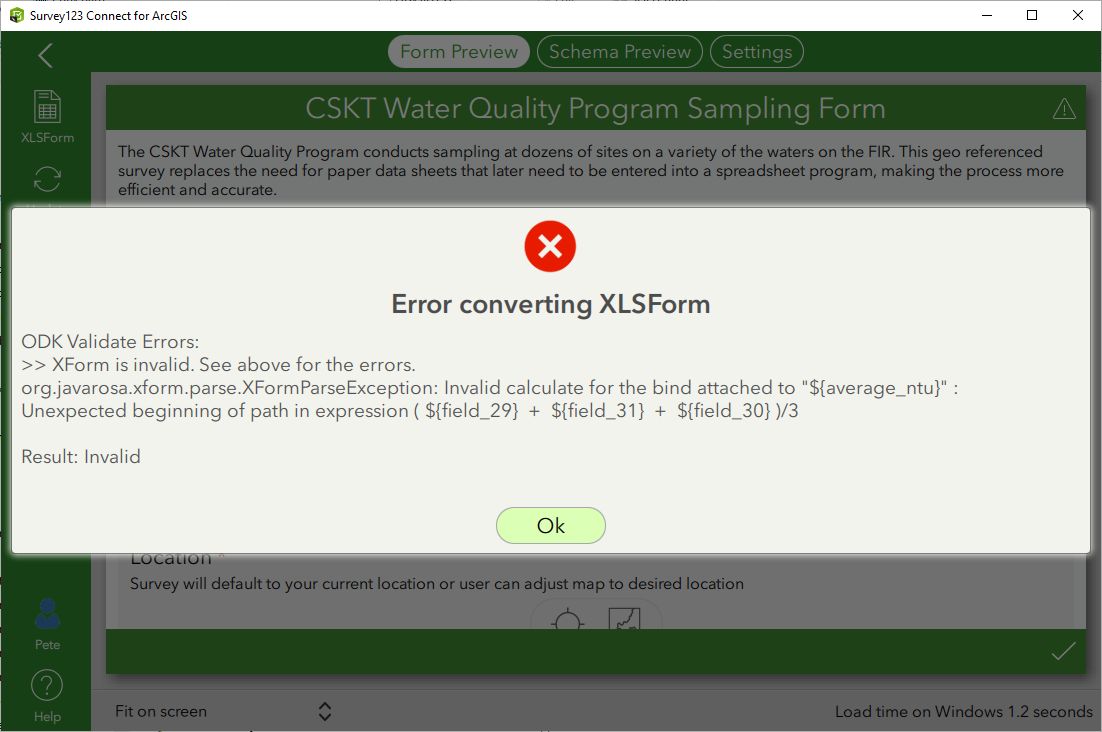# SURVEY 123 -Is there a way to have multiple numeric fields on the form, and the last one will sum/average/"Other math functions" the previous entries. Similarly if it's a percentage to assure the entries add up to 100%? Thanks in advance for any ideas

421
4
02-10-2020 02:01 PMNew Contributor III

Trying to average multiple numeric inputs on a Survey 123 form.

Tags (2)
1 Solution

Accepted SolutionsbyEsri Contributor

Sorry, try

(\${first_field} + \${second_field}+ \${third_field}) div 3

4 RepliesbyEsri Contributor

Hi Pete,

You can do this only if you're using Survey123 Connect (not the web version).

1. Create another row for your summary record, for example 'summary_field'.

2. Insert your equation in the calculation field of 'summary_field'. For example, to calculate the average of three other fields:

(\${first_field} + \${second_field}+ \${third_field}) div 3

For more examples and operators see:

Hope this helps,

UriNew Contributor III

Hi, i keep getting this message when trying to update the form. Tried the field to calculate as 'calculate' or 'decimal' neither worked. the formula is in the 'calculation' field as written. am i missing something? thanksbyEsri Contributor

Sorry, try

(\${first_field} + \${second_field}+ \${third_field}) div 3New Contributor III

Thanks! that did it!